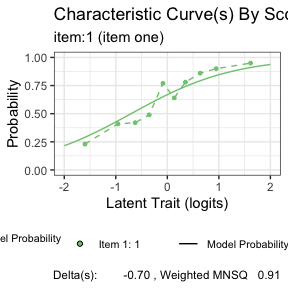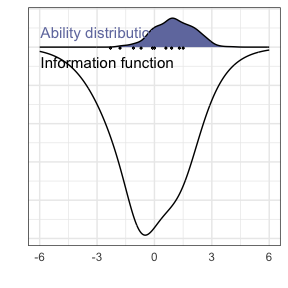# Introduction

conquestr has two main types of plots. The first is plots based on the plot command in ACER ConQuest. These plots are done using the generic function conquestr::plotRout The second is more general plots, usually of the inputs or outputs of IRT estimate.

# Example

## plotRout

library(conquestr)
myRout <- ConQuestRout()
plotRout(myRout)## General Plots

You can plot an arbitrary information function against a distribution of students - a so called information Wright map.

myDeltaDots <- data.frame(
id = c(1:10),
itemid = paste0("item", 1:10),
delta = rnorm(10)
)

MyTaus <- data.frame(
id = c(2L, 10L),
itemId = NA,
step = c(1L, 1L),
tau = rnorm(2)
)

informationWrightMap(myItems = myItemList, myAbilities = myPersons, minTheta = -6, maxTheta = 6)